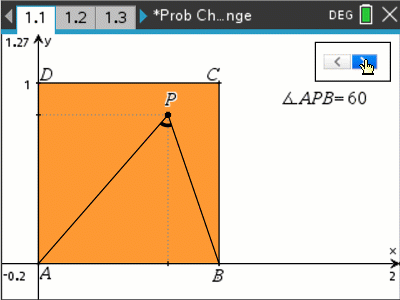# Activities

•• ##### AuthorAust Senior

50 Minutes

• ##### Device
•TI-Nspire™ CX
•TI-Nspire™ CX CAS

4.5

## Circular Probability#### Activity Overview

A triangle is formed such that two vertices are on the base of a unit square and the third vertex somewhere within the square. What is the probability that the angle at this third vertex will be greater than 90 degrees? A diagram helps to provide a visual, the interactive nature of the TI-nspire file makes it easy for students to explore, estimate the probability and determine a geometrical approach to solving the problem.

#### Objectives

Use area and geometry to determine the probability that the angle at the vertex is greater than 90 degrees if the triangle is formed such that two vertices are on the base of a unit square and the third vertex somewhere within the unit square.

• Area
• Sector
• Segment
• Thales law
• Vertices